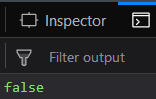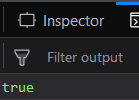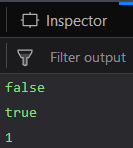# Underscore.js _.isNaN() Function

• Last Updated : 06 May, 2022

_.isNaN() function:

• It is used to find whether the value of the object passed is NaN or not.
• If the object’s value is NaN then the output will be true otherwise, it will be false.
• We can even perform addition, subtraction etc operations in this function.

Syntax:

`_.isNaN(object)`

Parameters:
It takes only one argument which is the object that needs to be checked.
Return value:
It returns true if the object’s value is NaN otherwise it returns false.
Examples:

• Passing a number to the _.isNan() function:
The _.isNaN() function takes the element that is passed to it and checks whether it’s value is NaN or not. Since a number is passed and we know that number have their own value so, the output will be false.

## html

 ```<``html``>`` ` `<``head``>``    ``<``script` `src` `=``"https://cdnjs.cloudflare.com/ajax/libs/underscore.js/1.9.1/underscore-min.js"` `>``    `````` ` `<``body``>``    ``<``script` `type``=``"text/javascript"``>``        ``var a=10;``        ``console.log(_.isNaN(10));``    `````` ` ``

Output:• Passing ‘NaN’ to the _.isNan() function:
Since this time, Nan itself is passed to the function, so, when the function checks then the it finds that the variable passed has NaN value. Ans therefore, the output will be true.

## html

 ```<``html``>``  ` `<``head``>``    ``<``script` `src` `=``"https://cdnjs.cloudflare.com/ajax/libs/underscore.js/1.9.1/underscore-min.js"` `>``    ``````  ` `<``body``>``    ``<``script` `type``=``"text/javascript"``>``        ``console.log(_.isNaN(NaN));``    ``````  ` ``

Output:• Passing “undefined” to _.isNaN() function:
The _.isNaN() function takes the parameter which here is “undefined” and starts checking. We know that, “Undefined” does not have any value and hence it’s value is definitely not NaN. Therefore, the answer is false.

## html

 ```<``html``>``  ` `<``head``>``    ``<``script` `src` `=``"https://cdnjs.cloudflare.com/ajax/libs/underscore.js/1.9.1/underscore-min.js"` `>``    ``````  ` `<``body``>``    ``<``script` `type``=``"text/javascript"``>``        ``console.log(_.isNaN(undefined));``    ``````  ` ``

Output:• Performing operations on the output of _.isNan() function:
Here, we are using the example 2 and 3 explained above. And then storing their value in variables ‘a’ and ‘b’. Therefore, the variable ‘a’ has false and ‘b’ has true. Finally we are performing an OR operation on both the ‘a’ and ‘b’ and storing the result in variable ‘c’. Since ‘b’ is true, therefore, ‘c’ is 1.

## html

 ```<``html``>``  ` `<``head``>``    ``<``script` `src` `=``"https://cdnjs.cloudflare.com/ajax/libs/underscore.js/1.9.1/underscore-min.js"` `>``    ``````  ` `<``body``>``    ``<``script` `type``=``"text/javascript"``>``        ``var a = _.isNaN(undefined);``        ``var b = _.isNaN(NaN);``        ``var c = a + b;``        ``console.log(a);``        ``console.log(b);``        ``console.log(c);``    ``````  ` ``

Output:NOTE:
These commands will not work in Google console or in firefox as for these additional files need to be added which they didn’t have added.
 ```<``script` `type``=``"text/javascript"` `src` `=``"https://cdnjs.cloudflare.com/ajax/libs/underscore.js/1.9.1/underscore-min.js"``>```